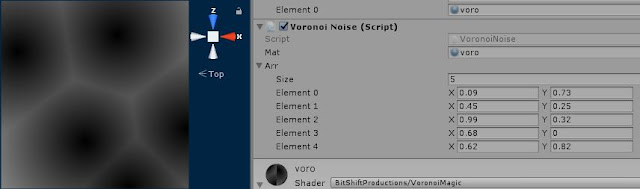### What is Voronoi ?

You may have heard of Voronoi noise if you have worked with software like blender or substance painter. It's really good way of making surfaces that look like they have sharp creases enclosing a smooth surface.
In this part we will see how to create a Voronoi pattern in Unity and how to set your own points in the shader itself to create the Voronoi pattern.
We will go over the 'Noise' part of Voronoi noise in Part 2.
The basic element that the Voronoi pattern depends is the 'Distance Field' function.
Take the case where there are 'N' points and we have to find the distance field of those points,
We have to calculate the distance between each pixel and the point closest to it.
This is what we will end up having in this tutorial:Voronoi Pattern Made With Given Points
We will be creating a script that passes a set of Vector2s to a shader which in turn draws it.
Before we get to C# scripting, we will see how to make the shader.
First of all we don't have any properties for this shader.😋. We will have properties in the coming parts.
But we do have a global variable called 'float2 _points' - This can be accessed through C# code.
Let's look the two structs that we have:
``````float2 _points;
struct appdata
{
float4 vertex : POSITION;
float2 uv : TEXCOORD0;
};

struct v2f
{
float2 uv : TEXCOORD0;
float4 vertex : SV_POSITION;
};
``````
No fancy-pancy stuff.
``````v2f vert (appdata v)
{
v2f o;
o.vertex = UnityObjectToClipPos(v.vertex);
o.uv = v.uv;
return o;
}
``````
Nothing special here either. Now the fragment shader:
``````fixed4 frag (v2f i) : SV_Target
{
fixed4 col = fixed4(0,0,0,1);
float minDist = 1.0;
float2 coord = i.uv;
for (int i = 0; i < 5; i++)
{
float dist = distance(coord, _points[i]);
minDist = min(minDist, dist);
}
col += minDist;
return col;
}
``````
We will see all the important parts and break it down.
``````for (int i = 0; i < 5; i++)
{
float dist = distance(coord, _points[i]);
minDist = min(minDist, dist);
}``````
Here we are iterating through all the points and keeping the minimum distance value in minDist.
Then after that we are just adding that as the colour of the pixel.
So we essentially just drew the distance field of those points.
What we have to do now... is that we have to access those points by C# code.
So the Unity API provides us a way to do so, with the Material.SetVectorArray function.
So here is the C# code:
``````using UnityEngine;
public class VoronoiNoise : MonoBehaviour
{
public Material mat;
public List<Vector2> points;

void Update ()
{
if(points.Count == 5)
mat.SetVectorArray("_points", ConvertToVec4(points));
}

List<Vector4> ConvertToVec4(List<Vector2> vec2)
{
List<Vector4> vec4 = new List<Vector4>();
for (int i = 0; i < vec2.Count; i++)